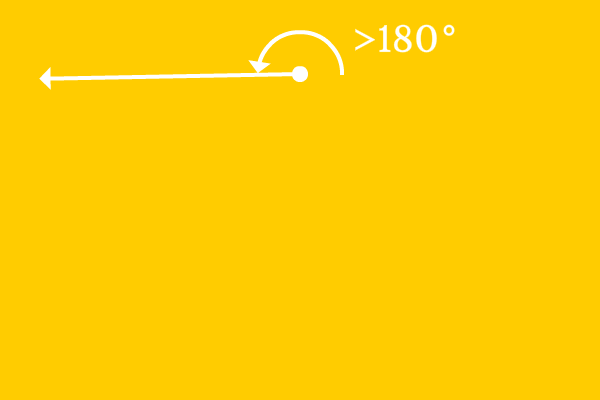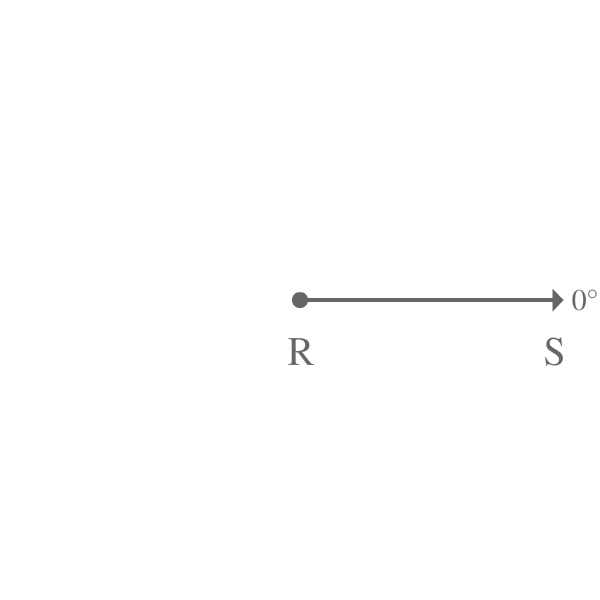# Reflex angle## Definition

An angle of more than $180^\circ$ but less than $360^\circ$ is called a Reflex angle.

The region from more than $180^\circ$ to less than $360^\circ$ is called reflex. If the angle lies in this region, the angle is known as the reflex angle geometrically.

### Case study

Geometrically, reflex angles are formed possibly in two different cases.

1

#### Reflex angle by a LineThe angle of a line is usually measured from $0^\circ$ angle. So, assume the ray $\overrightarrow{RS}$ is initially at zero degrees angle.

Later, the ray $\overrightarrow{RS}$ is rotated to $245^\circ$ angle in anticlockwise direction to reach new position where the ray $\overrightarrow{RS}$ is called as $\overrightarrow{RT}$.

In other words, the ray $\overrightarrow{RS}$ made an angle of $245^\circ$ to reach its final position from its initial position.

$\angle SRT = 245^\circ$

The angle $SRT$ is $245^\circ$ and it is greater than $180^\circ$ but less than $360^\circ$. Therefore, the $\angle SRT$ is called as a reflex angle.

If any straight line makes an angle of greater than $180^\circ$ and less than $360^\circ$ by the rotation, the angle is called as a reflex angle.

2

#### Reflex angle between two lines$U$ is a point where two rays $\overrightarrow{UV}$ and $\overrightarrow{UW}$ are started.

In this case, the ray $\overrightarrow{UV}$ becomes reference line to ray $\overrightarrow{UW}$ and vice-versa to measure angle between them.

The angle between them is measured as $310^\circ$ and it is denoted by $\angle VUW$.

$\angle VUW = 310^\circ$

The angle $VUW$ is greater than $180^\circ$ and less than $360^\circ$. Therefore $\angle VUW$ is called as reflex angle.

As example here, if angle between any two lines is greater than 180 and less than 360, the angle between them is an example to the reflex angle.

Latest Math Topics
Jun 26, 2023
Jun 23, 2023

###### Math Questions

The math problems with solutions to learn how to solve a problem.

Learn solutions

Practice now

###### Math Videos

The math videos tutorials with visual graphics to learn every concept.

Watch now

###### Subscribe us

Get the latest math updates from the Math Doubts by subscribing us.